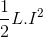# What are electrical power system parameters

## Electrical power system parameters

Let’s try to understand different parameters of electrical power system. Three basic parameters of electrical power system are electrical resistance, inductance and capacitance.

#### Resistance

The resistance is an inherent property of any material, due to which it resists the flow of current by obstructing the movement of electrons through it due to collision with stationary atoms. The heat generated due to this process is dissipated and known as ohmic power loss. While current flows through a resistor, there will not be any phase difference between the voltage and current, which means current and voltage are in same phase; the phase angle between them is zero. If I current flows through an electrical resistance R for t seconds, then total energy consumed by the resistor is I2.R.t. This energy is known as active energy and corresponding power is known as active power.

#### Inductance

Inductance is the property by virtue of which an inductor stores energy in a magnetic field during the positive half cycle and gives away this energy during the negative half cycle of single phase power supply. If a current ‘I’ flows through a coil of inductance L Henry, the energy stored in the coil in the form of a magnetic field is given byThe power associated with inductance is reactive power.

#### Capacitance

Capacitance is the property by virtue of which a capacitor stores energy in static electric field during positive half cycle and give away during negative half cycle of supply. The energy stored between two parallel metallic plates of electric potential difference V and capacitance across them C, is expressed asThis energy is stored in form of static electric field. The power associated with a capacitor is also reactive power.Scroll to Top
Scroll to Top